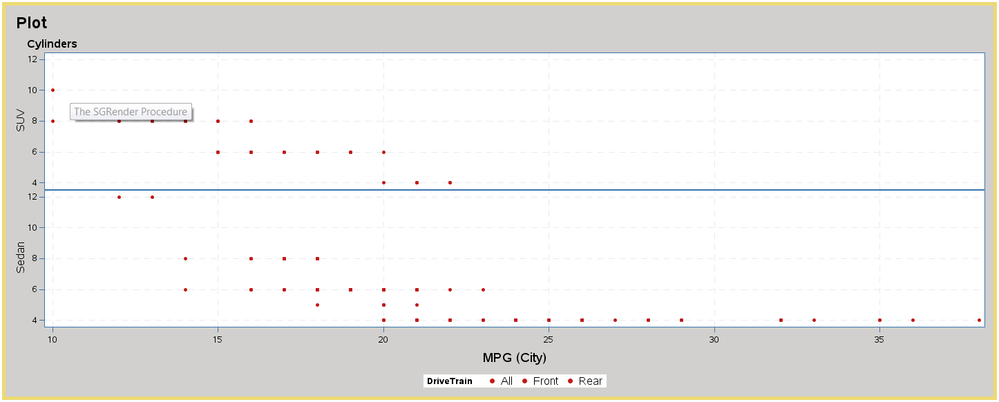## Some Questions about Layout Datalattice

Hi All,

I have three questions about layout data lattice.  I used sample dataset sashelp.cars to create the plot and attached it below.

1.  How to specify different colors and symbols for values of DriveTrain, "All", "Front", and "Rear"?

2. There are two panels named "SUV" and "Sedan" in the plot.  How to show their variable name "Type" on the top like "Cylinders"?

3. How to make the sidebar located at the right top corner of this plot?

I hope everything makes sense to you.  Thanks!

``````proc sql;
create table CARS as
select *
from sashelp.cars
where Type = "SUV" or Type = "Sedan";
quit;

proc template;
define statgraph scatter;
begingraph / backgroundcolor=CXD1D0CE border=true borderattrs=(color=CXEDDA74 thickness=5);
entrytitle halign=left textattrs=(size=15pt) "Plot";
layout datalattice rowvar=Type /
rowaxisopts=(display=standard griddisplay=on gridattrs=(pattern=dash) labelposition=top labelattrs=(size=11pt))
columnaxisopts=(griddisplay=on gridattrs=(pattern=dash) labelattrs=(size=12pt weight=normal))
layout prototype;
scatterplot x=MPG_City y=Cylinders / group=DriveTrain markerattrs=(color=CXC11B17 symbol=circlefilled) name='a';
endlayout;
sidebar / align=bottom spacefill=false;
discretelegend "a" / border=false valueattrs=(size=11pt) title="DriveTrain";
endsidebar;
endlayout;
endgraph;
end;
run;

proc sgrender data=CARS template=scatter;
run;``````1 ACCEPTED SOLUTION

Accepted SolutionsDanH_sas
SAS Super FREQ

## Re: Some Questions about Layout Datalattice

1.  How to specify different colors and symbols for values of DriveTrain, "All", "Front", and "Rear"?

[Dan] - If you care about which color and symbols are associated with each value, use a DiscreteAttrMap; otherwise, you can change the colors and symbols using the DATACONTRASTCOLORS and DATASYMBOLS options on the BEGINGRAPH statement.

2. There are two panels named "SUV" and "Sedan" in the plot.  How to show their variable name "Type" on the top like "Cylinders"?

[Dan] - Use a DATAPANEL with COLUMNS=1 (the default) instead of a DATALATTICE.

3. How to make the sidebar located at the right top corner of this plot?

[Dan] - There is not a nice way to do this. I would recommend keeping the legend at the bottom to optimize your space.

Below are the recommended changes to your code.

Hope this helps!

Dan

``````proc sql;
create table CARS as
select *
from sashelp.cars
where Type = "SUV" or Type = "Sedan";
quit;

proc template;
define statgraph scatter;
begingraph / backgroundcolor=CXD1D0CE border=true borderattrs=(color=CXEDDA74 thickness=5);
entrytitle halign=left textattrs=(size=15pt) "Plot";
discreteattrmap name="scatmap";
value "All" / markerattrs=(color=orange symbol=circlefilled);
value "Front" / markerattrs=(color=purple symbol=circlefilled);
value "Rear" / markerattrs=(color=green symbol=circlefilled);
enddiscreteattrmap;
discreteattrvar attrvar=mapvar var=Drivetrain attrmap="scatmap";
layout datapanel   classvars=(Type) /
rowaxisopts=(display=standard griddisplay=on gridattrs=(pattern=dash) labelposition=top labelattrs=(size=11pt))
columnaxisopts=(griddisplay=on gridattrs=(pattern=dash) labelattrs=(size=12pt weight=normal))
layout prototype;
scatterplot x=MPG_City y=Cylinders / group=mapvar name='a';
endlayout;
sidebar / align=bottom spacefill=false;
discretelegend "a" / border=false valueattrs=(size=11pt) title="DriveTrain";
endsidebar;
endlayout;
endgraph;
end;
run;

proc sgrender data=CARS template=scatter;
run;``````
2 REPLIES 2DanH_sas
SAS Super FREQ

## Re: Some Questions about Layout Datalattice

1.  How to specify different colors and symbols for values of DriveTrain, "All", "Front", and "Rear"?

[Dan] - If you care about which color and symbols are associated with each value, use a DiscreteAttrMap; otherwise, you can change the colors and symbols using the DATACONTRASTCOLORS and DATASYMBOLS options on the BEGINGRAPH statement.

2. There are two panels named "SUV" and "Sedan" in the plot.  How to show their variable name "Type" on the top like "Cylinders"?

[Dan] - Use a DATAPANEL with COLUMNS=1 (the default) instead of a DATALATTICE.

3. How to make the sidebar located at the right top corner of this plot?

[Dan] - There is not a nice way to do this. I would recommend keeping the legend at the bottom to optimize your space.

Below are the recommended changes to your code.

Hope this helps!

Dan

``````proc sql;
create table CARS as
select *
from sashelp.cars
where Type = "SUV" or Type = "Sedan";
quit;

proc template;
define statgraph scatter;
begingraph / backgroundcolor=CXD1D0CE border=true borderattrs=(color=CXEDDA74 thickness=5);
entrytitle halign=left textattrs=(size=15pt) "Plot";
discreteattrmap name="scatmap";
value "All" / markerattrs=(color=orange symbol=circlefilled);
value "Front" / markerattrs=(color=purple symbol=circlefilled);
value "Rear" / markerattrs=(color=green symbol=circlefilled);
enddiscreteattrmap;
discreteattrvar attrvar=mapvar var=Drivetrain attrmap="scatmap";
layout datapanel   classvars=(Type) /
rowaxisopts=(display=standard griddisplay=on gridattrs=(pattern=dash) labelposition=top labelattrs=(size=11pt))
columnaxisopts=(griddisplay=on gridattrs=(pattern=dash) labelattrs=(size=12pt weight=normal))
layout prototype;
scatterplot x=MPG_City y=Cylinders / group=mapvar name='a';
endlayout;
sidebar / align=bottom spacefill=false;
discretelegend "a" / border=false valueattrs=(size=11pt) title="DriveTrain";
endsidebar;
endlayout;
endgraph;
end;
run;

proc sgrender data=CARS template=scatter;
run;``````